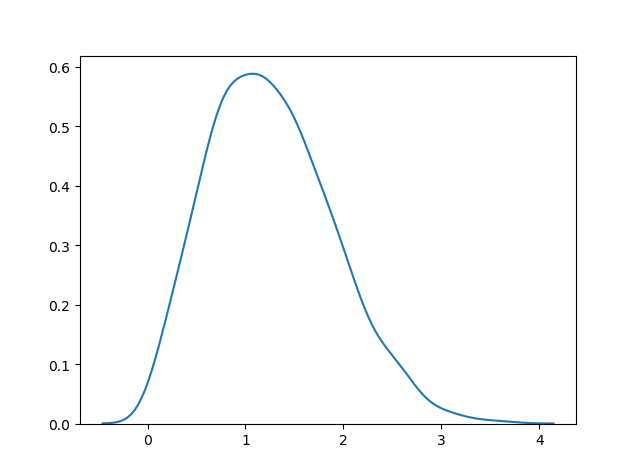# Rayleigh Distribution

## Rayleigh Distribution

Rayleigh distribution is used in signal processing.

It has two parameters:

`scale` - (standard deviation) decides how flat the distribution will be default 1.0).

`size` - The shape of the returned array.

### Example

Draw out a sample for rayleigh distribution with scale of 2 with size 2x3:

from numpy import random

x = random.rayleigh(scale=2, size=(2, 3))

print(x)
Try it Yourself »

## Visualization of Rayleigh Distribution

### Example

from numpy import random
import matplotlib.pyplot as plt
import seaborn as sns

sns.distplot(random.rayleigh(size=1000), hist=False)

plt.show()

### ResultTry it Yourself »

## Similarity Between Rayleigh and Chi Square Distribution

At unit stddev and 2 degrees of freedom rayleigh and chi square represent the same distributions.

W3Schools is optimized for learning and training. Examples might be simplified to improve reading and learning. Tutorials, references, and examples are constantly reviewed to avoid errors, but we cannot warrant full correctness of all content. While using W3Schools, you agree to have read and accepted our terms of use, cookie and privacy policy.